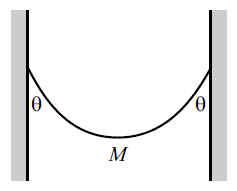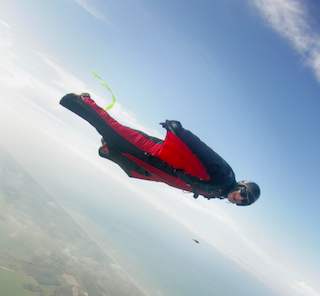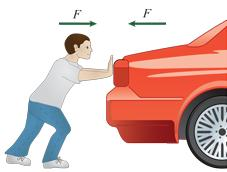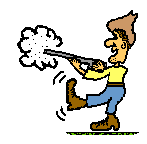Classical Mechanics

# Introduction to Forces: Level 2-3 Challenges

In the movie Spiderman 2, there's a famous minute or two long scene where Spiderman stops a train. If the initial speed of a five car out of control subway train was around the top speed of the NYC subway (27 m/s), and Spiderman stopped the train in exactly one minute, then what is the magnitude of the force in Newtons that Spiderman exerted on the train as he stopped it?

Details and Assumptions

• A standard New York City subway car full of terrified passengers has a mass of roughly 40,000 kg.
• You may assume the acceleration was constant and any other forces are negligible.A chain of mass 2 Kg hangs between two walls, with its ends at the same height. The chain makes an angle of $\theta=60^\circ$ with each wall, as shown in figure. Find the tension in the chain at the lowest point (mid-point). $(g=9.8\ SI units)$

###### This problem is a part of My Picks for JEE Mains 1Wingsuits allow humans to control fall and generate lift. The wingsuit flyer above controls his direction of flight by a direct application of which of Newton's laws?

###### Image credit: WikipediaYou push a heavy car by hand. The car, in turn, pushes back on you. Doesn't this mean that the forces cancel each other, making acceleration impossible?

Why or why not?

A. Yes: In accord with Newton's second law, $a =\frac{F_\text{net}}{m}$, and since $F_{net}=F_{you}+F_{car}=0$, no acceleration occurs.

B. Yes: In accord with Newton's first and third laws, an object continues in its state of rest unless it is compelled to change that state by forces impressed upon it. Since the net force is zero, the car doesn't move.

C. No: In accord with Newton's third law, I push the car and the car pushes with an equal and opposite force on me. Thus, with Newton's second law and $a=\frac{F}{m}$, the car accelerates, but so do I in an equal and opposite direction.

D. No: An external, horizontal force is applied to the car by my push; the car accelerates (in accord with Newton's first law).


Note: By acceleration we mean acceleration relative to the ground.

Problem credit: Conceptual Physics: Special Edition Series, Paul G. HewittMany people are familiar with the fact that a rifle recoils when fired. This recoil is the result of action-reaction force pairs. A gunpowder explosion creates hot gases that expand outward allowing the rifle to push forward on the bullet. Consistent with Newton's third law of motion, the bullet pushes backwards upon the rifle. Which of the following statements is true concerning the acceleration of the recoiling rifle?

×